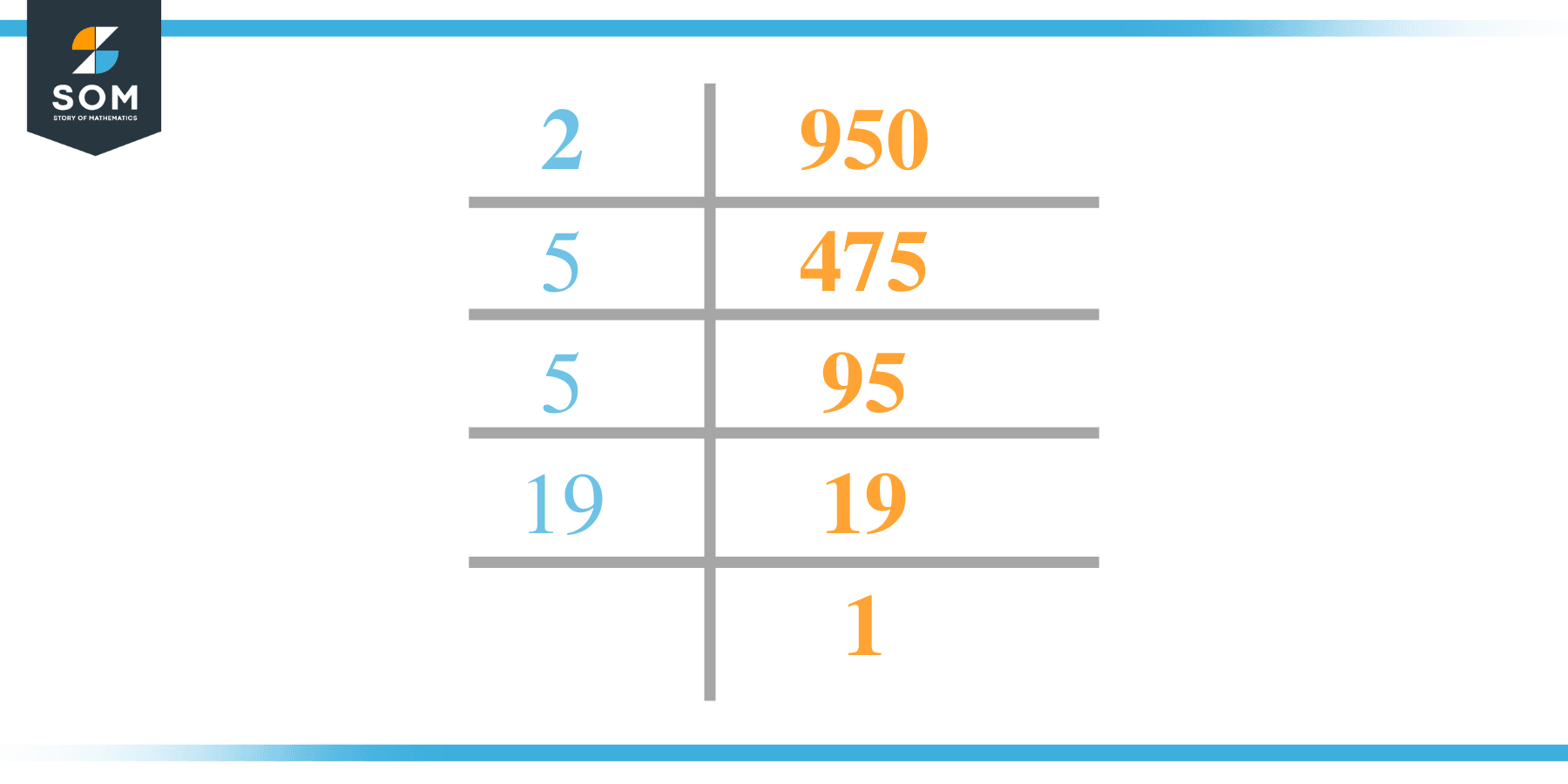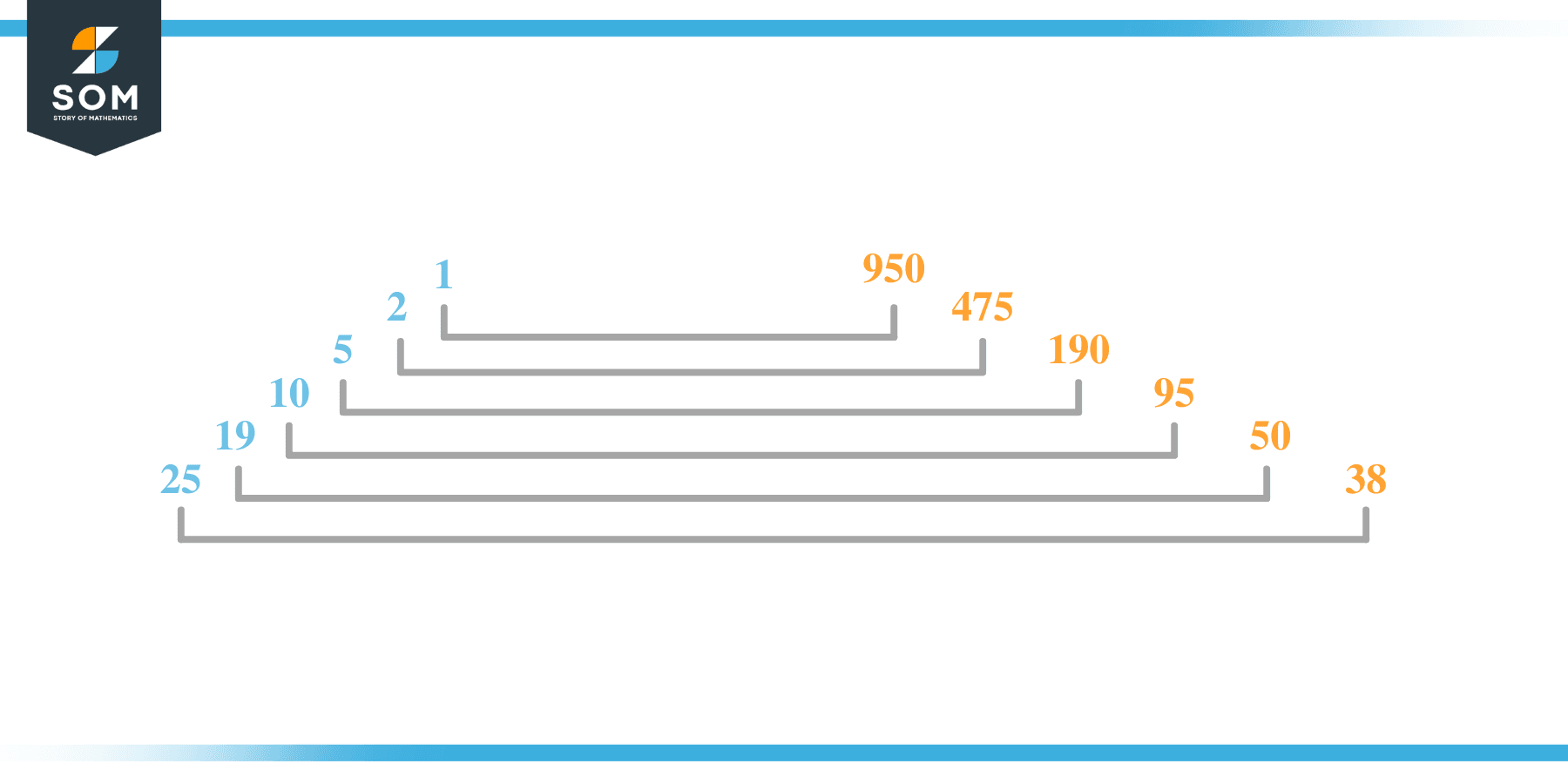# Factors of 950: Prime Factorization, Methods, and Examples

The factors of 950 are the listing of the integers that, when paired in groups and multiplied together, result in the number 950. The number 950 is an even composite, which indicates that it has more than two factors.Let us find out more.

### Factors of 950

Here are the factors of number 950.

Factors of 950: 1, 2, 5, 10, 19, 25, 38, 50, 95, 190, 475, and 950

### Negative Factors of 950

The negative factors of 950 are similar to their positive aspects, just with a negative sign.

Negative Factors of 950: –1, -2, -5, -10, -19, -25, -38, -50, -95, -190, -475, and -950

### Prime Factorization of 950

The prime factorization of 950 is the way of expressing its prime factors in the product form.

Prime Factorization: 2 x 5 x 5 x 19

In this article, we will learn about the factors of 950 and how to find them using various techniques such as upside-down division, prime factorization, and factor tree.

## What Are the Factors of 950?

The factors of 950 are 1, 2, 5, 10, 19, 25, 38, 50, 95, 190, 475, and 950. These numbers are the factors as they do not leave any remainder when divided by 950.

The factors of 950 are classified as prime numbers and composite numbers. The prime factors of the number 950 can be determined using the prime factorization technique.

## How To Find the Factors of 950?

You can find the factors of 950 by using the rules of divisibility. The divisibility rule states that any number, when divided by any other natural number, is said to be divisible by the number if the quotient is the whole number and the resulting remainder is zero.

To find the factors of 950, create a list containing the numbers that are exactly divisible by 950 with zero remainders. One important thing to note is that 1 and 950 are 950’s factors, as every natural number has 1 and the number itself as its factor.

1 is also called the universal factor of every number. The factors of 950 are determined as follows:

$\dfrac{950}{1} = 950$

$\dfrac{950}{2} = 475$

$\dfrac{950}{5} = 190$

$\dfrac{950}{10} = 95$

$\dfrac{950}{19} = 50$

$\dfrac{950}{25} = 38$

Therefore, 1, 2, 5, 10, 19, 25, 38, 50, 95, 190, 475, and 950 are the factors of 950.

### Total Number of Factors of 950

For 950, there are twelve positive factors and twelve negative ones. So in total, there are twenty-four factors of 950.

To find the total number of factors of the given number, follow the procedure mentioned below:

1. Find the factorization/prime factorization of the given number.
2. Demonstrate the prime factorization of the number in the form of exponent form.
3. Add 1 to each of the exponents of the prime factor.
4. Now, multiply the resulting exponents together. This obtained product is equivalent to the total number of factors of the given number.

By following this procedure, the total number of factors of 950 is given as:

The factorization of 950 is 1 x 2 x 5$^2$ x 19.

The exponent of 1, 2, and 19 is 1. The exponent of 5 is 2.

Adding 1 to each and multiplying them together results in 24.

Therefore, the total number of factors of 950 is 24. Twelve are positive, and twelve factors are negative.

### Important Notes

Here are some essential points that must be considered while finding the factors of any given number:

• The factor of any given number must be a whole number.
• The factors of the number cannot be in the form of decimals or fractions.
• Factors can be positive as well as negative.
• Negative factors are the additive inverse of the positive factors of a given number.
• The factor of a number cannot be greater than that number.
• Every even number has 2 as its prime factor, the smallest prime factor.

## Factors of 950 by Prime FactorizationThe number 950 is a composite. Prime factorization is a valuable technique for finding the number’s prime factors and expressing the number as the product of its prime factors.

Before finding the factors of 950 using prime factorization, let us find out what prime factors are. Prime factors are the factors of any given number that are only divisible by 1 and themselves.

To start the prime factorization of 950, start dividing by its most minor prime factor. First, determine that the given number is either even or odd. If it is an even number, then 2 will be the smallest prime factor.

Continue splitting the quotient obtained until 1 is received as the quotient. The prime factorization of 950 can be expressed as:

950 = 2 x 5 x 5 x 19

## Factors of 950 in PairsThe factor pairs are the duplet of numbers that, when multiplied together, result in the factorized number. Factor pairs can be more than one depending on the total number of factors given.

For 950, the factor pairs can be found as:

1 x 950 = 950

2 x 475 = 950

5 x 190 = 950

10 x 95 = 950

19 x 50 = 950

25 x 38 = 950

The possible factor pairs of 950 are given as (1, 950), (2, 475), (5, 190), (10, 95), (19, 50), and (25, 38).

All these numbers in pairs, when multiplied, give 950 as the product.

The negative factor pairs of 950 are given as:

-1 x -950 = 950

-2 x -475 = 950

-5 x -190 = 950

-10 x -95 = 950

-19 x -50 = 950

-25 x -38 = 950

It is important to note that in negative factor pairs, the minus sign has been multiplied by the minus sign, due to which the resulting product is the original positive number. Therefore, 1, -2, -5, -10, -19, -25, -38, -50, -95, -190, -475, and -950 are called negative factors of 950.

The list of all the factors of 950, including positive as well as negative numbers, is given below.

Factor list of 950: 1, -1, 2,  -2, 5, -5, 10, -10, 19, -19, 25, -25, 38, -38, 50, -50, 95, -95, 190, -190, 475, -475, 950, and -950

## Factors of 950 Solved Examples

To better understand the concept of factors, let’s solve some examples.

### Example 1

How many factors of 950 are there?

### Solution

The total number of Factors of 950 is twelve.

Factors of 950 are 1, 2, 5, 10, 19, 25, 38, 50, 95, 190, 475, and 950.

### Example 2

Find the factors of 950 using prime factorization.

### Solution

The prime factorization of 950 is given as:

950 $\div$ 2 = 475

475 $\div$ 5 = 95

95 $\div$ 5 = 19

19 $\div$ 19 = 1

So the prime factorization of 950 can be written as:

2 x 5 x 5 x 19 = 950